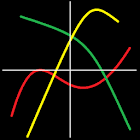Mathematics

All Android applications categories

All Android games categories# Mathematics

1.4K 7.6

7.6 Users
rating

## Screenshots

Description

This app will provide you a great tool if you want to plot a mathematic function.
You only have to write formula, and curve will be displayed in a customizable chart.
You can choose minimal and maximal abscissa (by default it is set from -5 to 5).
It is possible to display derivative function, so you can see both f(x) and f'(x) curves.
If you click on "VALUES" button, il shows all values between selected bounds.
You have a trace function that let you move a current point on curve, while its cordinates are displayed.
Likewise, tangent can be displayed on current point.
In addition, you can export the chart as an image and save it, or send it by mail.
It can replace your scientific calculator !
Enjoy !

Examples of possible functions :
f(x) = 5x+3
f(x) = 3x^2 (equivalent to 3x²)
f(x) = 4cos(π-x)
f(x) = tan(cos(x))
f(x) = ln(x+10)
f(x) = exp(-3x) (exp is exponential function)
f(x) = xsin(x) - 6/x

## Users review

from 1.403 reviews

"Great"

7.6